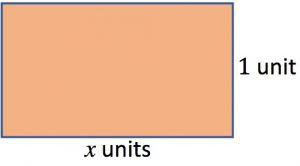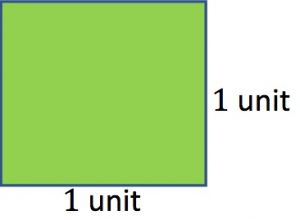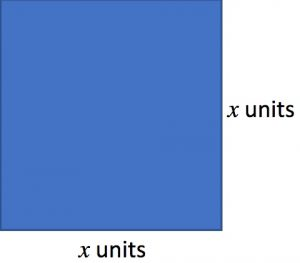1. You have 2 blue shapes, 3 green and 5 brown. Describe their properties.

2. Find the areas in square units of the 3 pieces blue, green and brown.3. Build one big geometrical shape using all 10 pieces, placing them edge to edge. Draw it, find its area and describe it in your notebook.

4. Build as many big shapes as you can using all 10 pieces. Find their areas in as many ways as you can. Make notes on what you notice about the areas of these geometrical shapes.

5. What mathematical relationship can you see between the different ways of calculating the area of the BIG rectangle? Explain how and why the areas found in different ways are equal.

6. If (2 + 3) is multiplied by (4 + 1), show how you would work out (2 + 3) (4 + 1) using a diagram.  Try different ways of coming up with the same answer.

7. Use your newly developed method to simplify the relationship you found between the different expressions for the area of the big rectangle.

Tagged with: